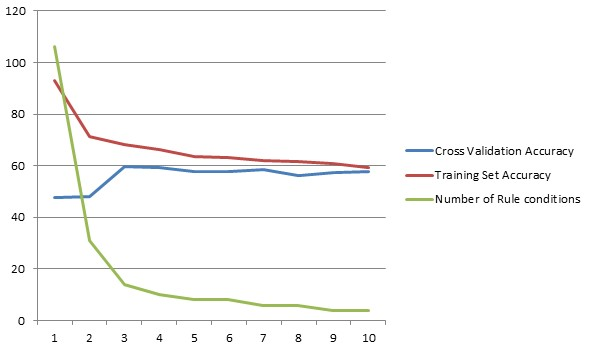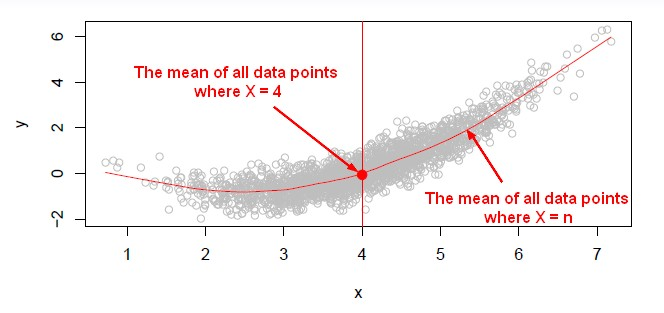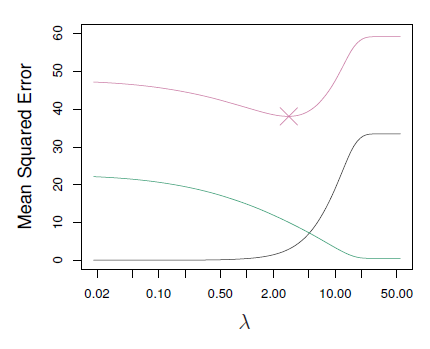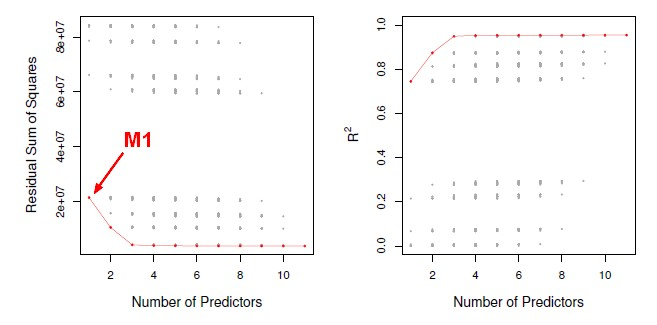# (Statistics|Data Mining) - (K-Fold) Cross-validation (rotation estimation)

Cross-validation, sometimes called rotation estimation is a resampling validation technique for assessing how the results of a statistical analysis will generalize to an independent new data set.

This is an extremely flexible and powerful technique and widely used approach in validation work for:

The measure of error for cross-validation is:

Otherwise, the cross-validation process is exactly the same for all type of problem.

## Procedure

### K stages

You divide at random the samples into K parts with a size about the same.

K-fold cross-validation will be done K times. In each stage, one fold gets to play the role of validation set whereas the other remaining parts (K-1) are the training set.

For each stage, cross-validation involves removing part of the data, then holding it out, fitting the model to the remaining part, and then applying the fitted model to the data that we've held out.

Stages:

• First Stage: The first part's the validation set. The other K-1 are the training parts as one big block.
• Second Stage: The second part is the validation set. The other K-1 are the training parts as one big block.
• K Stage: The K part is the validation set. The other K-1 are the training parts as one big block.

At the end, we'll summarize the test errors together to get an idea of the error. See just below cross-validation_error_estimate

A correct cross-validation procedure will possibly choose a different set of k variables for every fold in order to find the k variables most correlated with y. Why ? Because, in general, different training sets will disagree on which are the best k variables.

### Cross-validation error estimate

We take all the prediction errors from all K stages, we add them together, and that gives us what's called the cross-validation error rate.

Let the K parts be $C_1,C2, \dots, C_K$ where $C_k$ denotes the indices of the observations in part k. There are $n_k$ observations in part k: if N is a multiple of K, then $n_k = \frac{n}{K}$ .  $$\begin{array}{rrl} CV_{(K)} & = & \sum^K_{k=1} \frac{n_k}{n}\href{MSE}{MSE}_k \\ \text{where } MSE_k & = & \frac{ \displaystyle \sum_{i\in C_k}(y_i - \hat{y}_i)^2}{n_k} \end{array}$$ where:

• $\hat{y}_i$ is the fit for observation i, obtained from the data with fold k removed.
• the mean squared error is got by applying the fit to the K minus 1 parts that don't involve part number K. That gives us our fit $\hat{y}_i$ for observation i.
• we add up the error (MSE).
• we have a weighted average formula with $\frac{n_k}{n}$ because each of the folds might not be exactly the same size. If we are lucky that the n divides by k exactly, then that weight will just be 1/k.

Since this cross-validation error is just an average, the standard error of that average also gives us a standard error of the cross-validation estimate:

• We take the error rates from each of the folds.
• Their average is the cross-validation error rate.
• The standard error is the standard deviation of the cross-validation estimate.

The standard deviation is a useful estimate but not quite valid because we're computing the standard error as if the folds were independent observations but they're not strictly independent. $Error_k$ overlaps with, $Error_j$ because they share some training samples. There's some correlation between them. But it's still use because it's actually a quite good estimate, mathematically proved.

## Characteristic

Cross-validation uses every instance in the dataset as a test instance exactly once, instead of leaving the test set up to chance each time as for instance in a percentage split

## K

Picking K is also a bias variance trade-off for prediction error.

### LOOCV

Leave-one out cross-validation (LOOCV) is a special case of K-fold cross validation where the number of folds is the same number of observations (ie K = N).

There would be one fold per observation and therefore each observation by itself gets to play the role of the validation set. The other n minus 1 observations playing the role of training set.

With simple least-squares linear or polynomial regression, an amazing short-cut makes the performance cost of LOOCV the same as that of a single model. LOOCV represents a nice special case in the sense that this cross-validation can be done without actually having to refit the model at all. You do the fit on the overall data set in order to calculate the MSE.

$$CV_{(n)} = \frac{1}{n} \sum^n_{i=1} \left ( \frac{ \href{residual}{y_i - \hat{y}_i} }{ 1-h_i} \right ) ^2$$

where:

• $h_i$ tells you how much influence an observation has on its own fit. It's a number between 0 and 1 that punishes the residual, because it divides by a number that's small, and it inflates the residual.

### K as a bias-variance trade-off

For most of the methods and most statistical learning methods, K equals 5 or 10 tend to be a good choice, rather than have a leave-one out cross-validation.

In a leave-one out cross-validation, the n folds look very similar to each other, because the training sets are almost the same. They only differ by one observation and therefore are highly correlated. As the leave-one out cross-validation is actually trying to estimate the error rate for the training sample of almost the same size as what you have, it's got then a low bias but a high variance.

We get a curve that's got the minimum around 2 and it's pretty flat after that.

A 10-fold cross-validation shows the minimum around 2, but there's there's less variability than with a two-fold validation. They are more consistent because they're averaged together to give us the overall estimate of cross-validation.

So K equals 5 or 10-fold is a good compromise for this bias-variance trade-off.

## Biased

### Upward

One issue with cross-validation is that since the training set is not as big as the original training set, the estimates of prediction error will be biased up a little bit, because you have less data that you're working with.

leave-one out cross-validation has smaller bias in this sense, because the training set is almost the same in size as the original set but on the other hand, it's got higher variance, because the train sets that it's using are almost the same as the original set. They only differ by one observation.

### For ten-fold cross-validation

If we use ten-fold cross-validation as a means of model selection, the cross-validation estimate of test error is potentially biased upward, downward or unbiased.

There are competing biases: on one hand, the cross-validated estimate is based on models trained on smaller training sets than the full model, which means we will tend to overestimate test error for the full model.

On the other hand, cross-validation gives a noisy estimate of test error for each candidate model, and we select the model with the best estimate. This means we are more likely to choose a model whose estimate is smaller than its true test error rate, hence, we may underestimate test error. In any given case, either source of bias may dominate the other.

## Type

### Stratified

Stratified is a variant of the k-fold where you will ensure that each fold has the right proportion of each class value.

In “stratified” cross-validation, training and test sets have the same class distribution as the full dataset.

## Cross‐validation vs Others

Cross‐validation is better than repeated holdout (percentage split) as it reduces the variance of the estimate. stratified is even better and must be the standard.

Cross-validation explicitly separates the training set from the validation set in order to get a good idea of test error. With bootstrap method it's not the case, and that's cause a problem.

## Real Case

A real case with:

• 5,000 predictors,
• 50 samples.
• 2 classes outcome

Having more predictors than samples is, a more and more commonly occurring case

### Wrong Way

In order to build a simple classifier, we are following this procedure:

1. Predictor screening: We first select the best set of predictors based on the correlation with the outcome. Just find the 100 predictors having the largest correlation on their own with the class labels (the target label). Keep these predictors and throw the the remaining 4,900 predictors
2. Apply cross-validation in step two

Applying cross validation in step two, forgetting step one is a serious error. Cross-validation in this case can tell you that your classifier is perfect, when in fact your classifier is the same as flipping a coin. Why ?

• In Step 1, the procedure has already seen the labels of the training data, and made use of them. This is a form of training and must be included in the validation process.
• Using the full data set to choose the best variables means that we do not pay as much price as we should for overfitting (since we are fitting to the test and training set simultaneously). This will lead us to underestimate test error for every model size, but the bias is worst for the most complex models. Therefore, we are likely to choose a model that is more complex than the optimal model.

### Right Way

The right way is to apply cross-validation to both steps.

• We first define our folds, five folds cross-validation before we do any fitting, we remove one of the folds. And now we can do whatever we want on the other four parts. We can filter and fit however we want. When we've finished our fitting, we then take the model and we predict the response for the next part.
• So in each of the 4/5ths folds, we might screen off a different set of predictors each time. And we probably will.

And so that variability is going to get taken into account.

Key point being that we form the folds before we filter or fit to the data. So that we're applying cross-validation to the entire process, not just the second step.

## Documentation / Reference

Discover MoreData Mining - (Test|Expected|Generalization) Error

Test error is the prediction error that we incur on new data. The test error is actually how well we'll do on future data the model hasn't seen. The test error is the average error that results from using...Data Mining - (Missing Value|Not Available) NA

Is theresignificance in the fact that a value is missing? “Missing” means what … Unknown Unrecorded Irrelevant Most learning algorithms deal with missing values but they may make different...Machine Learning - (One|Simple) Rule - (One Level Decision Tree)

One Rule is an simple method based on a 1‐level decision tree described in 1993 by Rob Holte, Alberta, Canada. really simple so small/noisy/complex that nothing can be learned from them ...Machine Learning - K-Nearest Neighbors (KNN) algorithm - Instance based learning

“Nearest‐neighbor” learning is also known as “Instance‐based” learning. K-Nearest Neighbors, or KNN, is a family of simple: classification and regression algorithms based on Similarity...Model Building - ReSampling Validation

Resampling method are a class of methods that estimate the test error by holding out a subset of the training set from the fitting process, and then applying the statistical learning method to those held...R - Feature Selection - Model selection with Direct validation (Validation Set or Cross validation)

Feature selection through model generation and selection through a direct approach with : validation set and cross validation in R We are picking a subset of the observations and put them...R - K-fold cross-validation (with Leave-one-out)

Cross-validation in R. Leave-one-out cross-validation in R. Each time, Leave-one-out cross-validation (LOOV) leaves out one observation, produces a fit on all the other data, and then makes a...Statistics - (Shrinkage|Regularization) of Regression Coefficients

Shrinkage methods are more modern techniques in which we don't actually select variables explicitly but rather we fit a model containingall p predictors using a technique that constrains or regularizes...Statistics - Model Selection

Model selection is the task of selecting a statistical model from a set of candidate models through the use of criteria's Dimension reduction procedures generates and returns a sequence of possible...Statistics - Resampling through Random Percentage Split

Percentage Split (Fixed or Holdout) is a re-sampling method that leave out random N% of the original data. For example, you might select: 75% of the rows formed the training setfor building the model...